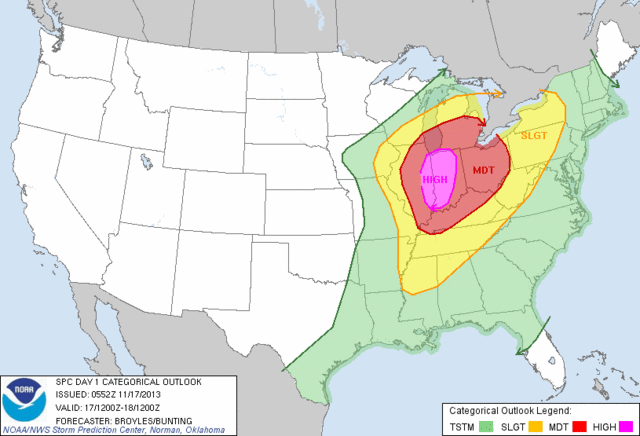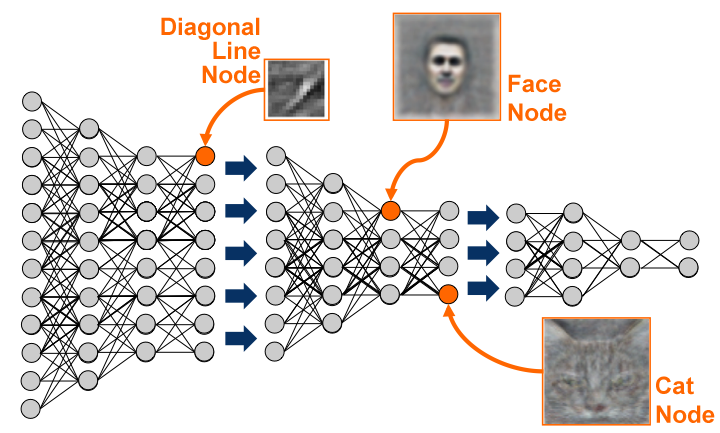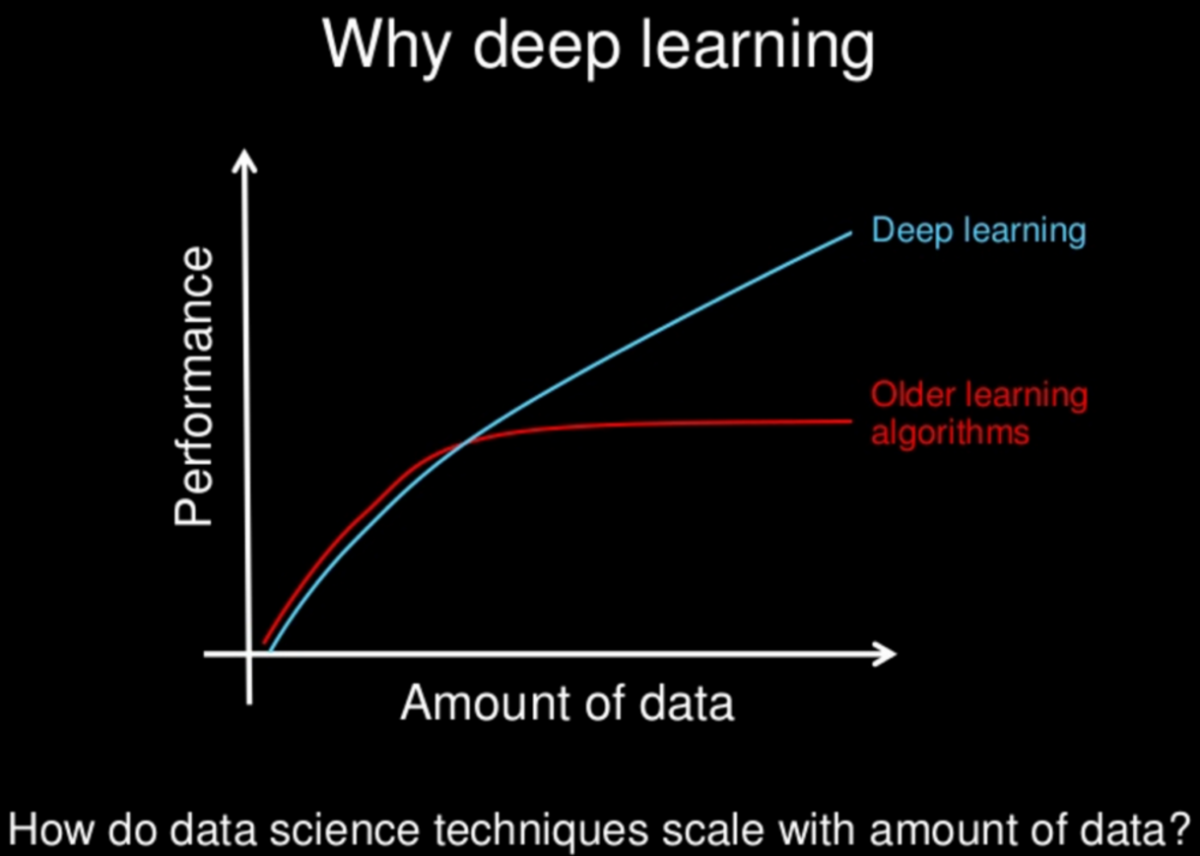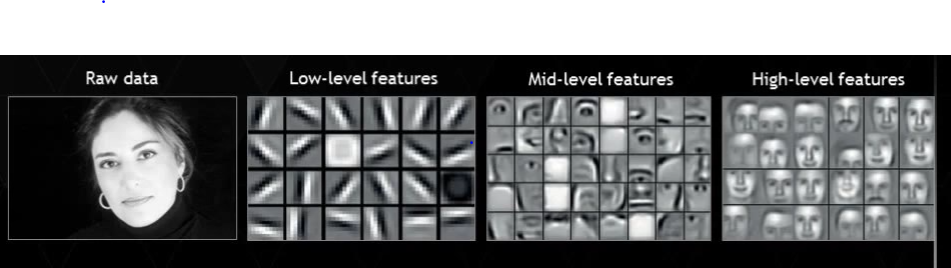Machine Learning Vs Deep Learning with examples and use case

For page specific messages
For page specific messages

What is Machine Learning?

Definition of Machine learning by Tom Mitchell best explains machine learning :

“A computer program is said to learn from experience E with respect to some class of tasks T and performance measure P if its performance at tasks in T, as measured by P, improves with experience E

Example 1 – Machine Learning – Predicting weights based on height Let us say you want to create a system which tells expected weight based on the height of a person. There could be several reasons why something like this could be of interest. You can use this to filter out any possible frauds or data capturing errors. The first thing you do is collect data. Let us say this is how your data looks like:Each point on the graph represents one data point. To start with we can draw a simple line to predict weight based on height. For example a simple line:

Weight (in kg) = Height (in cm) - 100

can help us make predictions. While the line does a decent job, we need to understand its performance. In this case, we can say that we want to reduce the difference between the Predictions and actuals. That is our way to measure performance.

Further, the more data points we collect (Experience), the better will our model become. We can also improve our model by adding more variables (e.g. Gender) and creating different prediction lines for them.

Example 2 – Storm prediction System

Let us take a slightly more complex example. Suppose you are building a storm prediction system. You are given the data of all the storms which have occurred in the past, along with the weather conditions three months before the occurrence of these storms.

Consider this, if we were to manually build a storm prediction system, what do we have to do?We have to first scour through all the data and find patterns in this data. Our task is to search which conditions lead to a storm.

We can either model conditions like – if the temperature is greater than 40-degree Celsius, humidity is in the range 80 to 100, etc. And feed these ‘features’ manually to our system.

Or else, we can make our system understand the data what will be the appropriate values for these features.

Now to find these values, you would go through all the previous data and try to predict if there will be a storm or not. Based on the values of the features set by our system, we evaluate how the system performs, viz how many times the system correctly predicts the occurrence of a storm. We can further iterate the above step multiple times, giving the performance as feedback to our system.

Let’s take our formal definition and try to define our storm prediction system: Our task ‘T’ here is to find what are the atmospheric conditions that would set off a storm. Performance ‘P’ would be, of all the conditions provided to the system, how many times will it correctly predict a storm. And experience ‘E’ would be the reiterations of our system.

What is Deep Learning?

The concept of deep learning is not new. It has been around for a couple of years now. But nowadays with all the hype, deep learning is getting more attention. As we did in Machine Learning, we will look at a formal definition of Deep Learning and then break it down with examples.

Deep learning is a particular kind of machine learning that achieves great power and flexibility by learning to represent the world as a nested hierarchy of concepts, with each concept defined in relation to simpler concepts, and more abstract representations computed in terms of less abstract ones.

Example 1 – Shape detection

Let me start with a simple example which explains how things happen at a conceptual level. Let us try and understand how we recognize a square from other shapes.The first thing our eyes do is check whether there are 4 lines associated with a figure or not (simple concept). If we find 4 lines, we further check, if they are connected, closed, perpendicular and that they are equal as well (nested hierarchy of concept).

So, we took a complex task (identifying a square) and broke it into simple less abstract tasks. Deep Learning essentially does this on a large scale.

Example 2 – Cat vs. Dog

Let’s take an example of an animal recognizer, where our system has to recognize whether the given image is of a cat or a dog.If we solve this as a typical machine learning problem, we will define features such as if the animal has whiskers or not, if the animal has ears & if yes, then if they are pointed. In short, we will define the facial features and let the system identify which features are more important in classifying a particular animal.

Now, deep learning takes this one step ahead. Deep learning automatically finds out the features which are important for classification, wherein Machine Learning we had to manually give the features. Deep learning works as follows:

Deep learning works as follows:

• It first identifies what are the edges that are most relevant to find out a Cat or a Dog
• It then builds on this hierarchically to find what combination of shapes and edges we can find. For example, whether whiskers are present, or whether ears are present, etc.
• After consecutive hierarchical identification of complex concepts, it then decides which of this features are responsible for finding the answer.

Comparison of Machine Learning and Deep Learning

Now that you have understood an overview of Machine Learning and Deep Learning, we will take a few important points and compare the two techniques.

Data dependencies

The most important difference between deep learning and traditional machine learning is its performance as the scale of data increases. When the data is small, deep learning algorithms don’t perform that well. This is because deep learning algorithms need a large amount of data to understand it perfectly. On the other hand, traditional machine learning algorithms with their handcrafted rules prevail in this scenario. Below image summarizes this fact.Hardware dependencies

Deep learning algorithms heavily depend on high-end machines, contrary to traditional machine learning algorithms, which can work on low-end machines. This is because the requirements of deep learning algorithm include GPUs which are an integral part of its working. Deep learning algorithms inherently do a large amount of matrix multiplication operations. These operations can be efficiently optimized using a GPU because GPU is built for this purpose.

Feature engineering

Feature engineering is a process of putting domain knowledge into the creation of feature extractors to reduce the complexity of the data and make patterns more visible to learning algorithms to work. This process is difficult and expensive in terms of time and expertise.

In Machine learning, most of the applied features need to be identified by an expert and then hand-coded as per the domain and data type.

For example, features can be pixel values, shape, textures, position and orientation. The performance of most of the Machine Learning algorithm depends on how accurately the features are identified and extracted.

Deep learning algorithms try to learn high-level features from data. This is a very distinctive part of Deep Learning and a major step ahead of traditional Machine Learning. Therefore, deep learning reduces the task of developing new feature extractor for every problem. Like, CNN (Convolutional Neutral Network)  will try to learn low-level features such as edges and lines in early layers then parts of faces of people and then the high-level representation of a face.Problem Solving approach

When solving a problem using traditional machine learning algorithm, it is generally recommended to break the problem down into different parts, solve them individually and combine them to get the result. Deep learning in contrast advocates to solve the problem end-to-end.

Let’s take an example to understand this.

Suppose you have a task of multiple object detection. The task is to identify what is the object and where is it present in the image.In a typical machine learning approach, you would divide the problem into two steps, object detection, and object recognition. First, you would use a bounding box detection algorithm like grabcut, to skim through the image and find all the possible objects. Then of all the recognized objects, you would then use object recognition algorithm like SVM with HOG to recognize relevant objects.

On the contrary, in deep learning approach, you would do the process end-to-end. For example, in a YOLO net (which is a type of deep learning algorithm), you would pass in an image, and it would give out the location along with the name of the object.

Execution time

Usually, a deep learning algorithm takes a long time to train. This is because there are so many parameters in a deep learning algorithm that training them takes longer than usual. State of the art deep learning algorithm ResNet takes about two weeks to train completely from scratch. Whereas machine learning comparatively takes much less time to train, ranging from a few seconds to a few hours.

This is turn is completely reversed on testing time. At test time, deep learning algorithm takes much less time to run. Whereas, if you compare it with k-nearest neighbors (a type of machine learning algorithm), test time increases on increasing the size of data. Although this is not applicable to all machine learning algorithms, as some of them have small testing times too.

Interpretability

Last but not the least, we have interpretability as a factor for comparison of machine learning and deep learning. This factor is the main reason deep learning is still thought 10 times before its use in industry.

Let’s take an example. Suppose we use deep learning to give automated scoring to essays. The performance it gives in scoring is quite excellent and it is the near-human performance. But there’s is an issue. It does not reveal why it has given that score. Indeed mathematically you can find out which nodes of a deep neural network were activated, but we don’t know what their neurons were supposed to model and what these layers of neurons were doing collectively. So we fail to interpret the results.

On the other hand, machine learning algorithms like decision trees give us crisp rules as to why it chose what it chose, so it is particularly easy to interpret the reasoning behind it. Therefore, algorithms like decision trees and linear/logistic regression are primarily used in industry for interpretability.

Where is Machine Learning and Deep Learning being applied right now?

The wiki article gives an overview of all the domains where machine learning has been applied. These include:

• Computer Vision: for applications like vehicle number plate identification and facial recognition.
• Information Retrieval: for applications like search engines, both text search, and image search.
• Marketing: for applications like automated email marketing, target identification
• Medical Diagnosis: for applications like cancer identification, anomaly detection
• Natural Language Processing: for applications like sentiment analysis, photo tagging